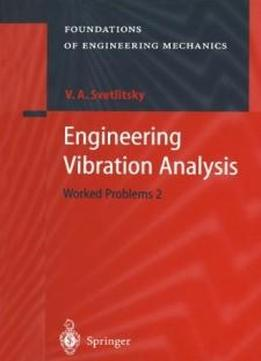# Engineering Vibration Analysis: Worked Problems 2 (foundations Of Engineering Mechanics) by Valery A. Svetlitsky / 2010 / English / PDF

Constantly increasing attention is paid in the course 'Vibration 'Theory' to vibration of mechanical systems with distributed parameters, since the real elements of machines, devices, and constructions are made of materials that are not perfectly rigid. 'Therefore, vibrations of the objects including, for ex­ ample, rod elastic elements excite the vibrations of these elements, which can produce a substantial effect on dynamic characteristics of moving objects and on readings of instruments. For a mechanical engineer working in the field of design of new technolo­ gies the principal thing is his know-how in developing the sophisticated math­ ematical models in which all specific features of operation of the objects under design in real conditions are meticulously taken into account. So, the main emphasis in this book is made on the methods of derivation of equations and on the algorithms of solving them (exactly or approximately) taking into con­ sideration all features of actual behavior of the forces acting upon elastic rod elements. 'The eigen value and eigen vector problems are considered at vibrations of curvilinear rods (including the rods with concentrated masses). Also consid­ ered are the problems with forced vibrations. When investigating into these problems an approximate method of numerical solution of the systems of lin­ ear differential equations in partial derivatives is described, which uses the principle of virtual displacements. Some problems are more complicated than others and can be used for practical works of students and their graduation theses.

Constantly increasing attention is paid in the course 'Vibration 'Theory' to vibration of mechanical systems with distributed parameters, since the real elements of machines, devices, and constructions are made of materials that are not perfectly rigid. 'Therefore, vibrations of the objects including, for ex­ ample, rod elastic elements excite the vibrations of these elements, which can produce a substantial effect on dynamic characteristics of moving objects and on readings of instruments. For a mechanical engineer working in the field of design of new technolo­ gies the principal thing is his know-how in developing the sophisticated math­ ematical models in which all specific features of operation of the objects under design in real conditions are meticulously taken into account. So, the main emphasis in this book is made on the methods of derivation of equations and on the algorithms of solving them (exactly or approximately) taking into con­ sideration all features of actual behavior of the forces acting upon elastic rod elements. 'The eigen value and eigen vector problems are considered at vibrations of curvilinear rods (including the rods with concentrated masses). Also consid­ ered are the problems with forced vibrations. When investigating into these problems an approximate method of numerical solution of the systems of lin­ ear differential equations in partial derivatives is described, which uses the principle of virtual displacements. Some problems are more complicated than others and can be used for practical works of students and their graduation theses.

views: 654